# 火星

Firestar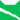雷族 (Q627)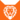狮族 (Q646)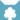天族 (Q632)[临时][注 1]雷族 武士雷族 副族长
（继任自虎星 (Q712)雷族 族长
（继任自蓝星 (Q713)狮族 族长天族 族长[临时][注 1]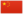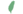拉斯特 Rusty  羅斯提
 火爪 Firepaw  火掌
 火心 Fireheart  火心
 火星 Firestar  火星

Q621：雷族公猫，原宠物猫，自《呼唤野性》登场的角色

## 细节

### 外貌

• 他是一只身形高大、:3章皮毛光滑:127厚实、:9:204祖母绿眼睛、:219亮火焰色:26的短毛公猫，:猫物表有着淡橙色的腹部、:110一对大耳朵:219和一条长尾巴。:52
• 生前，他的身上满是战疤。:19章他的身侧有一道疤痕，:257一只耳朵撕裂。:110
• 他的外貌和父亲杰克极为相似。:512

### 趣闻

• 火蕨的名字是为了纪念他。:86
• 外孙女烁皮的外貌和他极为相似。
• 他的挚友灰条曾提议为她起名烁火Sparkfire）以纪念他，但未能遂愿。:7章
• 外曾孙[注 2]焰心的前缀:1章和后缀:22章都是为了纪念他。
• 这令焰心非常不满，最后在他的抗争下，更改为夜心Nightheart）:22章
• 作为一名族长，他最喜欢的职责之一就是举行学徒仪式:86
• 他会在发表谈话前花很多时间进行准备。:1
• 在游戏《魔兽世界：巨龙时代》World of Warcraft: Dragonflight）的Beta版本中增加了数只猫NPC，包含橙色虎斑猫火心Fireheart）、棕色虎斑猫虎掌Tigerclaw）、银白虎斑猫银溪Silverstream）、黑白猫高尾Talltail）和灰猫黄牙Yellowfang）

## 登场书目

• 呼唤野性 (Q57)[主人公][主要角色][视角人物][首次登场🐱]
• 寒冰烈火 (Q59)[主人公][主要角色][视角人物]
• 疑云重重 (Q60)[主人公][主要角色][视角人物]
• 风起云涌 (Q65)[主人公][主要角色][视角人物]
• 险路惊魂 (Q66)[主人公][主要角色][视角人物]
• 力挽狂澜 (Q67)[主人公][主要角色][视角人物]
• 午夜追踪 (Q139)[配角]
• 新月危机 (Q140)[配角]
• 重现家园 (Q141)[配角]
• 星光指路 (Q142)[配角]
• 黄昏战争 (Q143)[配角]
• 日落和平 (Q144)[配角]
• 预视力量 (Q152)[视角人物]
• 暗河汹涌 (Q153)[配角]
• 驱逐之战 (Q154)[配角]
• 天蚀遮月 (Q155)[配角]
• 暗夜长影 (Q156)[配角]
• 拂晓之光 (Q157)[配角]
• 第四学徒 (Q158)[配角]
• 战声渐近 (Q159)[配角]
• 暗夜密语 (Q160)[配角]
• 月光印记 (Q161)[配角]
• 武士归来 (Q162)[配角]
• 群星之战 (Q163)[主要角色][死亡时刻✝]
• 学徒探索 (Q170)[配角]
• 雷影交加 (Q171)[对话提及]
• 天空破碎 (Q172)[配角]
• 极夜无光 (Q173)[配角]
• 烈焰焚河 (Q174)[对话提及]
• 风暴来袭 (Q175)[视角人物]
• 静默冰融 (Q3579)[对话提及]
• 叠影重障 (Q3624)[对话提及]
• 暗由心生 (Q4183)[配角]
• 无星之地 (Q4385)[对话提及]
• 迷雾明光 (Q4621)[主要角色][视角人物]
• 河翻浪涌 (Q4643)[对话提及]
• 天穹将倾 (Q4799)[对话提及]
• 火星的探索 (Q176)[主人公][主要角色][视角人物]
• 蓝星的预言 (Q177)[配角]
• 天族的命运 (Q178)[对话提及]
• 钩星的承诺 (Q179)[间接提及]
• 黄牙的秘密 (Q180)[配角]
• 高星的复仇 (Q181)[配角]
• 黑莓星的风暴 (Q182)[视角人物]
• 蛾飞的幻象 (Q183)[仅于幻象]
• 鹰翅的旅程 (Q184)[对话提及]
• 鸦羽的拷问 (Q186)[对话提及]
• 松鼠飞的希冀 (Q3561)[配角]
• 灰条的誓言 (Q4057)[对话提及]
• 豹星的荣耀 (Q4426)[配角]
• 冬青叶的故事 (Q187)[对话提及]
• 雾星的征兆 (Q188)[配角]
• 虎掌的愤怒 (Q190)[配角]
• 叶池的祈愿 (Q191)[配角]
• 鸽翅的沉默 (Q192)[配角]
• 鹅羽的诅咒 (Q194)[仅于幻象]
• 乌爪的告别 (Q195)[对话提及]
• 蛾翅的秘密 (Q3628)[配角]
• 黛西的至亲 (Q3629)[对话提及]
• 斑毛的叛变 (Q4337)[对话提及]
• 黑脚的清算 (Q4336)[配角]
• 长鞭崛起 (Q206)[配角]
• 河族阴云 (Q4311)[配角]
• 变革之风 (Q4411)[配角]
• 逐出影族 (Q4769)[配角]
• 武士失踪 (Q207)[仅于回忆]
• 武士避难 (Q208)[对话提及]
• 武士回归 (Q209)[配角]
• 和平破碎 (Q213)[仅于回忆]
• 族群救星 (Q214)[配角]
• 武士之心 (Q215)[配角]
• 紧急救援 (Q216)[对话提及]
• 洪水过后 (Q218)[对话提及]
• 族群的秘密 (Q199)[追溯修订]
• 族群的猫 (Q200)[配角]
• 族群的守则 (Q201)[配角]
• 族群的战争 (Q202)[配角]
• 终极指南 (Q204)[配角]
• 族群决定 (Q4580)[配角]
• 日落之后：正确的选择？ (Q4323)[配角]
• 长老的忧虑 (Q4324)[对话提及]
• 超越守则：光灵的恻隐之心 (Q4321)[配角]
• ## 周边

更多资料：火星/周边

【系列1】火星和叶星
• 系列1——火星和叶星、松鸦羽和灰条、虎星褐皮
• 勇敢的拉斯特抛弃了宠物猫的安稳舒适，捍卫雷族，并在之后作为传奇的火星来领导他们；同时适应性强的叶星——过去曾是不属于任何族群的泼皮猫的她——在天族重建后接下了其领导权。这些谦逊而辉煌的族长总是捍卫他们的族群。
• 长约7厘米。
• 一组约12.99美金；整套三组约38.97美金。

## 外部链接

### 官方文章

• 火之传奇 （专题）
• 族群中的领导者们 （提及）
• 猫武士长篇外传：无限世界 （提及）
• 名垂青史的族长们 （专题）
• 一路走来－《风暴来袭》 （隐射）
• 《迷失群星》诞生记 （提及）
• 角色聚光灯－黛西 （提及）
• 叶星：母亲还是族长？ （提及）
• 向猫妈妈致敬 （隐射）
• 欢迎来到第五族群 （提及）
• 选个绒毛娃娃：第二回 （投票选项）
• 第一场九命仪式 （专题）
• 感恩的行为 （提及）
• 消失的传统 （提及）
• 不容忽视的“爪字辈”们 （提及）
• 脱颖而出 （专题）
• 角色聚光灯－松鼠飞 （提及）
• 我们捕猎为生 （提及）
• 守则式微？ （提及）
• 武士守则：过时的遗俗？ （提及）
• 守则破坏者－《破灭守则》头脑风暴 （提及）
• 邪恶永存 （提及）
• 至尊反派 （提及）
• 创造反派 （提及）
• 团结则存，分裂则亡 （提及）
• 黎明星的荒唐：若和平不能给出答案 （提及）
• 远古猫 （提及）
• 共同的纽带 （提及）
• 族群还是至亲？ （提及）
• 预料之外的剧情 （提及）
• 你至亲的至亲 （提及）
• 我们的边界之外 （提及）
• 解读征兆 （提及）
• 写个好预言 （提及）
• 得不到星辰祝福的爱侣们 （提及）
• 生命诚可贵，爱情价更高 （隐射）
• 禁忌之爱 （提及）
• 改变终将降临 （提及）
• 自一切伊始 （提及）
• 巫医的天职 （提及）
• 原谅与改正 （提及）
• 沉重的要求 （提及）
• 角色聚光灯－豹星 （提及）
• 《预言开始》主要角色盘点 （专题）
• 急水部落 （提及）
• 跨越边界 （提及）
• 命运指引着我们的脚掌…… （提及）
• 《新预言》主要角色盘点 （提及）
• 再访灰条 （提及）
• 《灰条的誓言》诞生记 （提及）
• 揭开猫武士大本营的面纱 （提及）
• 追忆往昔 （提及）
• 一切的开始 （专题）
• 蜡毛：星族的疏漏？ （提及）
• 《三力量》主要角色盘点 （提及）
• 血缘之外 （提及）
• 真正的武士？ （隐射）
• 抉择的问题 （提及）
• 族群是怎么开始的 （提及）
• 角色与薛定谔的家谱树 （提及）
• 绝妙的地图 （提及）
• 建立一个宗教 （提及）
• 《无星之地》：粉丝圈里人气最高的剧情理论 （提及）
• The Forest Map: ThunderClan （提及）
• 介绍你们选出的2021绒毛娃娃 （隐射）
• 最重要的是…… （提及）
• 泥掌的叛变 （提及）
• 河族的回归 （提及）
• The Forest Map: RiverClan （提及）
• 艾琳·亨特的意见：我们需要谈谈雪绺 （提及）
• 《预言开始》的族长们 （提及）
• 何谓族群？ （提及）
• 致敬鬃霜 （隐射）
• 来自作者维琪·霍姆斯的独家新章节 （隐射）
• 我们所铭记的死亡 （提及）
• 《猫武士》世界中的死亡 （提及）
• 当火焰大放异彩 （专题）
• 于我而言—一切的伊始 （提及）
• 一路走来－《迷雾明光》 （隐射）
• 《河翻浪涌》：粉丝圈里人气最高的剧情理论 （隐射）
• 夜心和传承的重担 （提及）
• 灵魂、勇气和忠诚 （提及）
• 寒冷北风：细看夜星的生命与领导 （提及）
• 武士的誓词 （提及）
• The Forest Map: WindClan （提及）
• 火星的五大鼠脑子时刻 （专题）
• 一路走来－《河翻浪涌》 （提及）
• Erin Hunter video special: Exclusive anniversary interview （提及）
• 何谓英雄？ （提及）
• 灰爪的五大逗趣时刻 （提及）
• 一日为宠物猫，终身为宠物猫 （提及）
• 换族者：他们会留下吗？ （提及）
• 一路走来－《天穹将倾》 （提及）
• 《影瘴迷心》：粉丝圈里人气最高的剧情理论 （提及）
• 云尾的五大《预言开始》时刻 （提及）
• 何谓反派？ （提及）
• 亮心与《雷族神偷》 （提及）
• 《预言开始》趣闻！ （提及）
• 《预言开始》中的五大族长 （专题）
• 武士名号 （提及）
• 火心的五大必知之事 （专题）

## 脚注

1. 原因：天族重建 (Q3676) - 在《火星的探索》中，火星和沙风重建现代天族的过程
2. 夜心是他的外孙女烁皮的儿子。
3. 他的武士美德之一是力量strength），在正体中文版中错译为“胆识”。

## 参考文献

这篇文章基于CC BY-SA 3.0许可使用了猫武士维基（英语）Firestar一文中的部分内容。